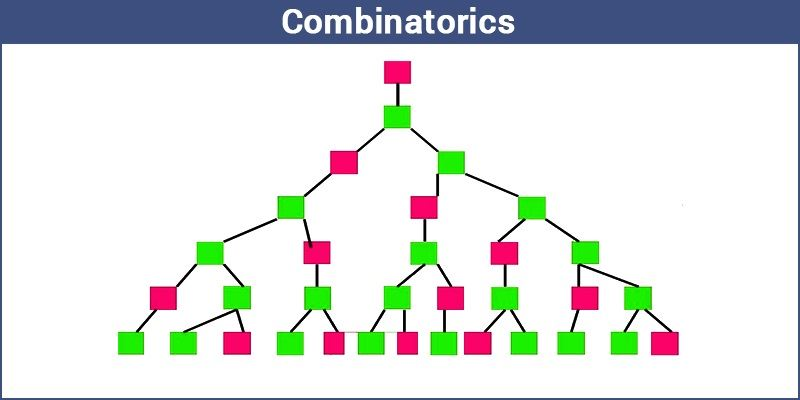# Combinatorics

Combinatorics is a stream of mathematics that concerns the study of finite discrete structures. It deals with the study of permutations and combinations, enumerations of the sets of elements. It characterizes Mathematical relations and their properties.

Mathematicians uses the term “Combinatorics” as it refers to the larger subset of Discrete Mathematics.  It is frequently used in computer Science to derive the formulas and it is used for the estimation of the analysis of the algorithms. In this article, let us discuss what is combinatorics, its features, formulas, applications and examples in detail.

## Features of combinatoricsSome of the important features of the combinatorics are as follows:

• Counting the structures of the provided kind and size.
• To decide when particular criteria can be fulfilled and analyzing elements of the criteria, such as combinatorial designs.
• To identify “greatest”, “smallest” or “optimal” elements, known as external combinatorics.

Combinatorial structures that rise in an algebraic concept, or applying algebraic techniques to combinatorial problems, known as algebraic combinatorics.

## What are permutation and Combination?

In English, we make use of the word “combination” without thinking if the order is important. Let’s take a simple instance.

The fruit salad is a combination of grapes, bananas, and apples. The order of fruits in the salad does not matter because it is the same fruit salad.

But, let us assume that, the combination of a key is 475. You need to take care of the order, since the other combinations like 457, 574, or others won’t work. Only the combination of 4 – 7 – 5 can unlock.

Hence, to be precise;

• When the order does not have much impact, it is said to be a combination.
• When the order does have an impact, it is said to be a permutation.

## Combinatorics Formulas

The mathematical form of Permutation and Combination:

Permutation Formula:

Permutation: The act of an arranging all the members of a set into some order or sequence, or rearranging the ordered set, is called the process of permutation.

Mathematically Permutation is given as

k-permutation of n is:

$$\begin{array}{l}^{n}P_{k} = \frac{n!}{(n-k)!}\end{array}$$

Combination Formula

Combination: Selection of members of the set where the order is disregarded.

k-combination of n is:

$$\begin{array}{l}^{n}C_{k} = \frac{n!}{k!.(n-k)!}\end{array}$$

## Applications of combinatorics

Combinatorics is applied in most of the areas such as:

• Communication networks, cryptography and network security
• Computational molecular biology
• Computer architecture
• Scientific discovery
• Languages
• Pattern analysis
• Simulation
• Databases and data mining
• Homeland security
• Operations research

## Combinatorics Solved Examples

Example 1: Arrange the letters of the word TAMIL so that

• T is always next to L
• T and L are always together

Solution:

Let’s consider LT as one letter and keep it together. Now we have 4 alphabets that can be arranged in a row in

$$\begin{array}{l}^{4}P_{4}\end{array}$$
= 24. (General formula, nPr)

L and T can be interchanged in their positions in 2! Ways. Therefore, total arrangements is given by 4!2! = 48.

Example 2: Calculate the number of ways a cricket eleven can be selected out of a batch of 15 players if;

• no restriction on the selection.
• A specific player is always selected.
• A specific player is never chosen.

Solution:

• When there is no restriction on the selection. This means 15c11 gives you the total number of ways.
• Since a specific player is selected always. Therefore, 15 -1, we have 14c10
• Since a specific player is never selected, we have 14c11

Example 3: Calculate the number of committees of 5 students that can be chosen from a class of 25.

Solution:

Since we have to select 5 out of 25. Therefore,

$$\begin{array}{l}^{25}C^{5}= \frac{25 \times 24 \times 23 \times 22 \times 21 }{5 \times 4 \times 3 \times 2 \times 1}\end{array}$$

= 53130

### Combinatorics and Probability

In probability theory, there are many applications of combinatorics. For example, when we find the probability of occurrence of a particular event A, we can use the below formula:

P(A) = Probability that A occurs = Number of outcomes where A happen/Total number of possible outcomes

We can also use combinatorics to calculate the total number of possible outcomes.

Example 4: Four students namely P, Q, R, and S sit randomly at four corners of the classroom while playing a game. Find the probability that Q sits at the North-east corner of the room.

Solution:
We know that there are 24 ways that a student can sit at four corners. Also, there are 6 (i.e. 3! ways) possible ways that Q can sit at the North-east corner.
Therefore, the probability that Q sits at the North-east corner of the room
= Number of ways that Q can sit at the North-east corner/Total number of ways
= 6/24 = 1/4

To solve more Combinatorics problems with solutions involving Combinatorics formulas, you can visit and download BYJU’S – The Learning App from the Google Play store.